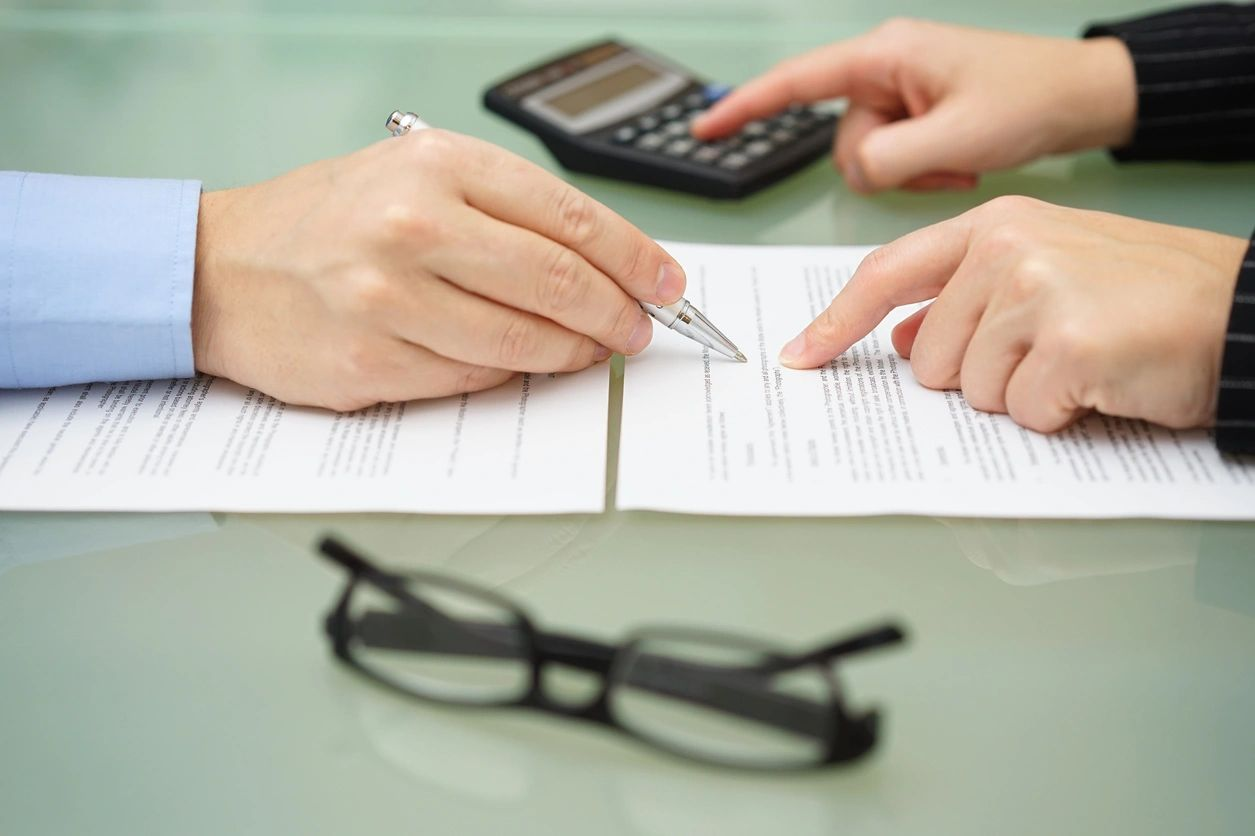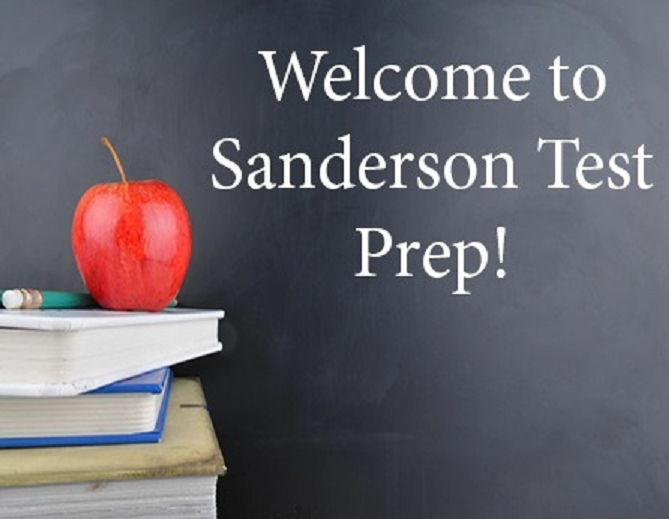# Free Geometry Content

Basics of Geometry

• Points, Lines, and Planes
• Segments
• Midpont Formula
• Distance Formula
• Perimeter and Area of Simple Figures
• Measuring Angles
• Angle Pairs

Reasoning and Proof

• Conditional Statements
• Inductive and Deductive Reasoning
• Using Postulates and Diagrams
• Algebraic Reasoning
• Proving Statements About Segments and Angles
• Proving Geometric Relationships

Parallel and Perpendicular Lines

• Pairs of Lines and Angles
• Parallel Lines and Transversals
• Proofs with Parallel Lines
• Proofs with Perpendicular Lines
• Equations of Parallel and Perpendicular Lines

Transformations

• Translations
• Reflections
• Rotations
• Congruence and Transformations
• Dilations
• SimilarityCongruent Triangles

• Angles of Triangles
• Congruent Polygons
• Side Angle Side (SAS)
• Equilateral and Isosceles
• Side Side Side (SSS)
• Angle Side Angle (ASA)
• Angle Angle Side (AAS)
• Using Congruent Triangles
• Coordinate Proofs

Relationships Within Triangles

• Perpendicular and Angle Bisectors
• Bisectors of Triangles
• Medians and Altitudes of Triangles
• The Triangle Midsegment Theorem
• Indirect Proof and Inequalities in One Triangle
• Inequalities in Two Triangles

• Angles of Polygons
• Properties of Parallelograms
• Proofs with Parallelograms
• Special Parallelograms
• Trapezoids and Kites

Similarity

• Similar Polygons
• Similarity via Angle Angle (AA)
• Similarity via Side Side Side (SSS) and Side Angle Side (SAS)
• Proportionality Theorems

Right Triangles and Pythagorean Theorem

• Pythagorean Theorem
• Special Right Triangles
• Similar Right Triangles
• Tangent Ratio
• Sine and Cosine Ratios
• Solving Right Triangles
• Law of Sines and Cosines

Circles

• Lines and Segments That Intersect Circles
• Finding Arc Measures
• Using Chords
• Inscribed Angles and Polygons
• Angle relationships in Circles
• Segment Relationships in Circles
• Circles in the Coordinate Plane

Circumference, Area, and Volume

• Circumference and Arc Length
• Areas of Circles and Sectors
• Areas of Polygons
• 3D Figures
• Volumes of Prisms and Cylinders
• Volumes of Pyramids
• Surface Area and Volume of Cones
• Surface Area and Volume of Spheres

Probability

• Intro to Probability
• Independent and Dependent
• Two-way Tables
• Disjoint and Overlapping Events
• Permutations and Combinations
• Binomial Distributions